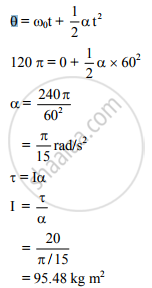# A Body Starts Rotating from Rest. Due to a Couple of 20 Nm It Completes 60 Revolutions in One Minute. Find the Moment of Inertia of the Body. - Physics

A body starts rotating from rest. Due to a couple of 20 Nm it completes 60 revolutions in one minute. Find the moment of inertia of the body.

#### Solution

T = 20 N m, n = 60 revolutions in 60 s = 1 r.p.s., t = 1 min = 60 s
Moment of inertia (I) = ?

Angular displacement θ = ωt = 2πnt
= 2π × 1 × 60∴ Moment of inertia of the body is 95.48 kg m2

Concept: Physical Significance of M.I (Moment of Inertia)
Is there an error in this question or solution?
2013-2014 (October)

Share